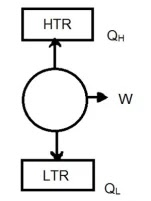# State the Second law of thermodynamics.

Question 04: State the Second law of thermodynamics.

Answer: Second Law of Thermodynamics: This law states that, where there is a definite limit to the amount of mechanical energy which can be obtained from a given quantity of the heat energy.

Kelvin- Plank statement of second law:

“It is impossible to build a cyclic engine that converts thermal energy into work with 100% efficiency”.From the figure, QH – QL = W.

From the statement, QH = W, QL = 0

But this is impossible.

Clausius Statement of Second law:

“It is impossible for an engine working in a cyclic process, to transfer heat from a body at a lower temperature to a body at a higher temperature without the aid of an external work done”.In figure,

Basically Q– Q= W, But

From the statement Q=Q; W = 0 But it is impossible.

Here, H.T.R = Higher Temperature Reservoir.

L.T.R = Lower Temperature Reservoir.

Rate this post#### By Mithu Khan

I am a blogger and educator with a passion for sharing knowledge and insights with others. I am currently studying for my honors degree in mathematics at Govt. Edward College, Pabna.